6. RHF 核坐标梯度的 U 矩阵计算¶

6.1. 准备工作¶

:
%matplotlib notebook

from pyscf import gto, scf, dft, lib
import numpy as np
from functools import partial
import warnings
from matplotlib import pyplot as plt
from pyxdh.Utilities import NucCoordDerivGenerator, DipoleDerivGenerator, NumericDiff

np.einsum = partial(np.einsum, optimize=["greedy", 1024 ** 3 * 2 / 8])
np.allclose = partial(np.allclose, atol=1e-6, rtol=1e-4)
np.set_printoptions(5, linewidth=150, suppress=True)
warnings.filterwarnings("ignore")
:
mol = gto.Mole()
mol.atom = """
O  0.0  0.0  0.0
O  0.0  0.0  1.5
H  1.0  0.0  0.0
H  0.0  0.7  1.0
"""
mol.basis = "6-31G"
mol.verbose = 0
mol.build()
:
<pyscf.gto.mole.Mole at 0x7f62b0731730>
:
:
:
scf_eng = scf.RHF(mol)
config = {
"scf_eng": scf_eng,
}
:

:

• H_1_ao $$h_{\mu \nu}^\mathbb{A}$$, H_1_mo $$h_{pq}^\mathbb{A}$$

• S_1_ao $$S_{\mu \nu}^\mathbb{A}$$, S_1_mo $$S_{pq}^\mathbb{A}$$

• eri1_ao $$(\mu \nu | \kappa \lambda)^\mathbb{A}$$, eri1_mo $$(pq|rs)^\mathbb{A}$$

:
def to_natm_3(mat: np.ndarray):
shape = list(mat.shape)
shape = [int(shape / 3), 3] + shape[1:]
return mat.reshape(shape)
:

6.2. 数值导数求取 U 矩阵¶

6.2.1. 没有经过“轨道旋转”的 U 矩阵¶

$\frac{\partial C_{\mu p}}{\partial \mathbb{A}} = C_{\mu m} U_{m p}^\mathbb{A}$

$U_{mp}^\mathbb{A} = (\mathbf{C}^{-1})_{m \mu} \frac{\partial C_{\mu p}}{\partial \mathbb{A}}$

:
nd_C.shape
:
(4, 3, 22, 22)

:
nd_U_1_nr = np.einsum("mu, Atup -> Atmp", np.linalg.inv(C), nd_C)
nd_U_1_nr.shape
:
(4, 3, 22, 22)

:
fig, ax = plt.subplots(figsize=(4, 3))
ax.hist(abs(nd_U_1_nr.ravel() - gradh_nr.U_1.ravel()), bins=np.logspace(np.log10(1e-9), np.log10(1e-1), 50), alpha=0.5)
ax.set_xscale("log")
fig.tight_layout()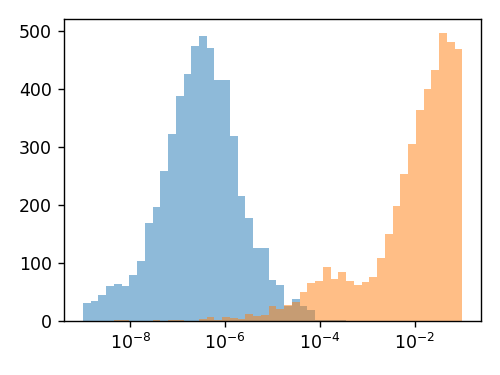6.2.2. 经过“轨道旋转”的 U 矩阵 (1) $$\mathscr{U}_{pq}^{A_t}$$ 的定义¶

:
fig, ax = plt.subplots(figsize=(4, 3))
ax.hist(abs(nd_U_1_nr.ravel() - gradh.U_1.ravel()), bins=np.logspace(np.log10(1e-9), np.log10(1e-1), 50), alpha=0.5)
ax.set_xscale("log")
fig.tight_layout()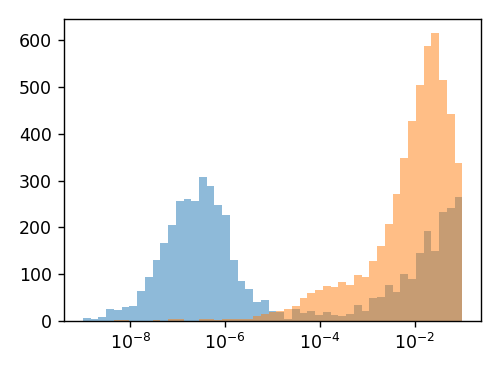$\begin{split}\begin{equation} \mathscr{U}_{pq}^{A_t} = \left\{ \begin{matrix} -\frac{1}{2} S_{ij}^{A_t} &\quad \textsf{occ-occ block} \\ -\frac{1}{2} S_{ab}^{A_t} &\quad \textsf{vir-vir block} \\ U_{ai}^{A_t} &\quad \textsf{vir-occ block} \\ U_{ia}^{A_t} &\quad \textsf{occ-vir block} \\ \end{matrix} \right. \end{equation}\end{split}$

\begin{split}\begin{align} U_{pq}^{A_t} + U_{qp}^{A_t} + S_{pq}^{A_t} &= 0 \\ \mathscr{U}_{pq}^{A_t} + \mathscr{U}_{qp}^{A_t} + S_{pq}^{A_t} &= 0 \end{align}\end{split}

6.2.3. 经过“轨道旋转”的 U 矩阵 (2) $$\mathscr{U}_{pq}^\mathbb{A}$$ 与 $$U_{pq}^\mathbb{A}$$ 的关系¶

$\mathscr{C}_{\mu p'} = C_{\mu p} X_{pp'}$

$\boldsymbol{\mathscr{C}} = \mathbf{C} \mathbf{X}$

• 为保证旋转后的系数矩阵仍然具有 $$D_{\mu \nu} = \mathscr{C}_{\mu i'} \mathscr{C}_{\nu i'} = C_{\mu i} C_{\nu i}$$ 的密度矩阵特性，$$\mathbf{X}$$ 必须是对角块矩阵 (block-diagonal)；或者说，$$X_{ai'} = 0$$$$X_{ia'} = 0$$

• $$\mathbf{X}$$ 需要是一个正交矩阵，即 $$\mathbf{X}^\dagger \mathbf{X} = \mathbf{1}$$，即 $$\mathbf{X}^\dagger = \mathbf{X}^{-1}$$

$\boldsymbol{\mathscr{C}}^\dagger \mathbf{S} \boldsymbol{\mathscr{C}} = \mathbf{X}^\dagger \mathbf{C}^\dagger \mathbf{S} \mathbf{C} \mathbf{X} = \mathbf{X}^\dagger \mathbf{1} \mathbf{X} = \mathbf{1}$

$\frac{\partial \boldsymbol{\mathscr{C}}}{\partial \mathbb{A}} = \boldsymbol{\mathscr{C}} \tilde{\boldsymbol{\mathscr{U}}}^\mathbb{A}$

$\boldsymbol{\mathscr{C}} \tilde{\boldsymbol{\mathscr{U}}}^\mathbb{A} = \mathbf{C} \mathbf{U}^\mathbb{A} \mathbf{X} + \mathbf{C} \partial_\mathbb{A} \mathbf{X}$

$\mathbf{X} \tilde{\boldsymbol{\mathscr{U}}}^\mathbb{A} \mathbf{X}^\dagger = \mathbf{U}^\mathbb{A} + (\partial_\mathbb{A} \mathbf{X}) \mathbf{X}^\dagger$

$\boldsymbol{\mathscr{U}}^\mathbb{A} = \mathbf{X} \tilde{\boldsymbol{\mathscr{U}}}^\mathbb{A} \mathbf{X}^\dagger = \mathbf{U}^\mathbb{A} + (\partial_\mathbb{A} \mathbf{X}) \mathbf{X}^\dagger$

$\mathscr{U}_{pq}^\mathbb{A} + \mathscr{U}_{qp}^\mathbb{A} + S_{pq}^\mathbb{A} = 0$

\begin{split}\begin{align} \mathbf{0} &= \frac{\partial \boldsymbol{\mathscr{C}}^\dagger}{\partial \mathbb{A}} \mathbf{S} \boldsymbol{\mathscr{C}} + \boldsymbol{\mathscr{C}}^\dagger \frac{\partial \mathbf{S}}{\partial \mathbb{A}} \boldsymbol{\mathscr{C}} + \boldsymbol{\mathscr{C}}^\dagger \mathbf{S} \frac{\partial \boldsymbol{\mathscr{C}}}{\partial \mathbb{A}} \\ &= \tilde{\boldsymbol{\mathscr{U}}}^{\mathbb{A} \dagger} \boldsymbol{\mathscr{C}}^\dagger \mathbf{S} \boldsymbol{\mathscr{C}} + \boldsymbol{\mathscr{C}}^\dagger \mathbf{S} \boldsymbol{\mathscr{C}} \tilde{\boldsymbol{\mathscr{U}}}^{\mathbb{A}} + \boldsymbol{\mathscr{C}}^\dagger \mathbf{S}^\mathbb{A} \boldsymbol{\mathscr{C}} \\ &= \tilde{\boldsymbol{\mathscr{U}}}^{\mathbb{A} \dagger} + \tilde{\boldsymbol{\mathscr{U}}}^{\mathbb{A}} + \boldsymbol{\mathscr{C}}^\dagger \mathbf{S}^\mathbb{A} \boldsymbol{\mathscr{C}} \end{align}\end{split}

$\tilde{\boldsymbol{\mathscr{U}}}^\mathbb{A} = \mathbf{X}^\dagger \boldsymbol{\mathscr{U}}^\mathbb{A} \mathbf{X}$

$\mathbf{0} = \mathbf{X}^\dagger \boldsymbol{\mathscr{U}}^{\mathbb{A} \dagger} \mathbf{X} + \mathbf{X}^\dagger \boldsymbol{\mathscr{U}}^{\mathbb{A}} \mathbf{X} + \mathbf{X}^\dagger \mathbf{C}^\dagger \mathbf{S}^\mathbb{A} \mathbf{C} \mathbf{X}$

$\mathbf{0} = \boldsymbol{\mathscr{U}}^{\mathbb{A} \dagger} + \boldsymbol{\mathscr{U}}^{\mathbb{A}} + \mathbf{C}^\dagger \mathbf{S}^\mathbb{A} \mathbf{C}$

$\begin{split}\begin{equation} \mathscr{U}_{pq}^{A_t} = \left\{ \begin{matrix} -\frac{1}{2} S_{ij}^{A_t} &\quad \textsf{occ-occ block} \\ -\frac{1}{2} S_{ab}^{A_t} &\quad \textsf{vir-vir block} \\ U_{ai}^{A_t} &\quad \textsf{vir-occ block} \\ U_{ia}^{A_t} &\quad \textsf{occ-vir block} \\ \end{matrix} \right. \end{equation}\end{split}$

:
fig, ax = plt.subplots(figsize=(4, 3))
ax.set_xscale("log")
fig.tight_layout()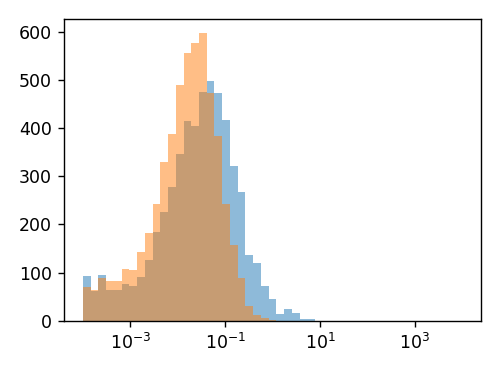$\mathbf{0} = \frac{\partial \mathbf{1}}{\partial \mathbb{A}} = \frac{\partial (\mathbf{X} \mathbf{X}^\dagger)}{\partial \mathbb{A}} = (\partial_\mathbb{A} \mathbf{X}) \mathbf{X}^\dagger + \mathbf{X} (\partial_\mathbb{A} \mathbf{X}^\dagger)$

$\boldsymbol{\mathscr{C}} = \mathbf{C}$

$\mathbf{X} = \mathbf{1}$

$\partial_\mathbb{A} \mathbf{X} = \boldsymbol{\mathscr{U}}^\mathbb{A} - \mathbf{U}^\mathbb{A}$

6.3. Fock 矩阵导数与 A 张量¶

6.3.1. Fock 矩阵导数 (1) Skeleton 导数¶

$F_{\mu \nu} = h_{\mu \nu} + (\mu \nu | \kappa \lambda) D_{\kappa \lambda} - \frac{1}{2} (\mu \kappa | \nu \lambda) D_{\kappa \lambda}$

$\frac{\partial F_{\mu \nu}}{\partial A_t} \leftarrow F_{\mu \nu}^{A_t} = h_{\mu \nu}^{A_t} + (\mu \nu | \kappa \lambda)^{A_t} D_{\kappa \lambda} - \frac{1}{2} (\mu \kappa | \nu \lambda)^{A_t} D_{\kappa \lambda}$
:
F_1_ao = (
+ H_1_ao
+ np.einsum("Atuvkl, kl -> Atuv", eri1_ao, D)
- 0.5 * np.einsum("Atukvl, kl -> Atuv", eri1_ao, D)
)
F_1_ao.shape
:
(4, 3, 22, 22)

:
:
True

$F_{pq}^{A_t} = C_{\mu p} F_{\mu \nu}^{A_t} C_{\nu q}$
:
F_1_mo = np.einsum("up, Atuv, vq -> Atpq", C, F_1_ao, C)
F_1_mo.shape
:
(4, 3, 22, 22)

:
nd_F_0_ao.shape
:
(12, 22, 22)
:
fig, ax = plt.subplots(figsize=(4, 3))
ax.hist(abs(nd_F_0_ao.ravel() - F_1_ao.ravel()), bins=np.logspace(np.log10(1e-9), np.log10(1e1), 50), alpha=0.5)
ax.hist(abs(nd_F_0_ao.ravel()), bins=np.logspace(np.log10(1e-9), np.log10(1e1), 50), alpha=0.5)
ax.set_xscale("log")
fig.tight_layout()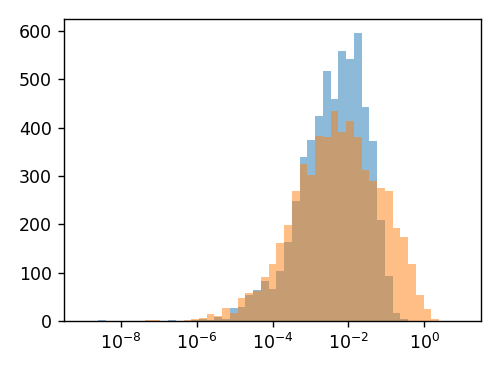6.3.2. Fock 矩阵导数 (2) U 导数与原子轨道下的 A 张量¶

\begin{split}\begin{align} \frac{\partial F_{\mu \nu}}{\partial A_t} &\leftarrow (\mu \nu | \kappa \lambda) \frac{\partial D_{\kappa \lambda}}{\partial A_t} - \frac{1}{2} (\mu \kappa | \nu \lambda) \frac{\partial D_{\kappa \lambda}}{\partial A_t} \\ &= 2 \left( (\mu \nu | \kappa \lambda) - \frac{1}{2} (\mu \kappa | \nu \lambda) \right) \big( C_{\kappa m} C_{\lambda i} + C_{\kappa i} C_{\lambda m} \big) U_{mi}^{A_t} \\ &= \big( 4 (\mu \nu | \kappa \lambda) - (\mu \kappa | \nu \lambda) - (\mu \lambda | \kappa \nu) \big) C_{\kappa m} C_{\lambda i} U_{mi}^{A_t} \end{align}\end{split}

$A_{\mu \nu, \kappa \lambda} = 4 (\mu \nu | \kappa \lambda) - (\mu \kappa | \nu \lambda) - (\mu \lambda | \kappa \nu)$

$\frac{\partial F_{\mu \nu}}{\partial A_t} \leftarrow A_{\mu \nu, \kappa \lambda} C_{\kappa m} C_{\lambda i} U_{mi}^{A_t}$

$\frac{\partial F_{\mu \nu}}{\partial A_t} = F_{\mu \nu}^{A_t} + A_{\mu \nu, \kappa \lambda} C_{\kappa m} C_{\lambda i} U_{mi}^{A_t}$

:
A_0_ao = 4 * eri0_ao - eri0_ao.swapaxes(-2, -3) - eri0_ao.swapaxes(-1, -3)
A_0_ao.shape
:
(22, 22, 22, 22)

:
F_U_ao = np.einsum("uvkl, km, li, Atmi -> Atuv", A_0_ao, C, Co, to_natm_3(gradh_nr.U_1)[:, :, :, so])

:
fig, ax = plt.subplots(figsize=(4, 3))
ax.hist(abs(nd_F_0_ao.ravel() - (F_1_ao + F_U_ao).ravel()), bins=np.logspace(np.log10(1e-11), np.log10(1e-1), 50), alpha=0.5)
ax.hist(abs(nd_F_0_ao.ravel()), bins=np.logspace(np.log10(1e-11), np.log10(1e-1), 50), alpha=0.5)
ax.set_xscale("log")
fig.tight_layout()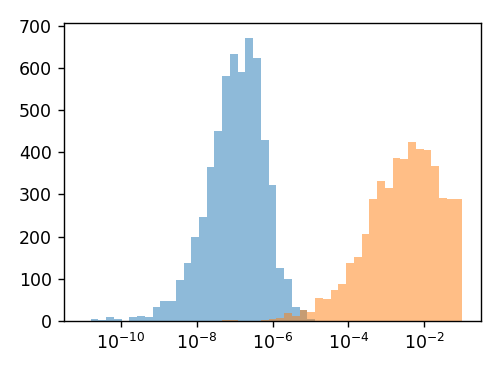6.3.3. Fock 矩阵导数 (3) 分子轨道下的 A 张量¶

$A_{pq, rs} = C_{\mu p} C_{\nu q} A_{\mu \nu, \kappa \lambda} C_{\kappa r} C_{\lambda s}$

:
A_0_mo = np.einsum("up, vq, uvkl, kr, ls -> pqrs", C, C, A_0_ao, C, C)
A_0_mo.shape
:
(22, 22, 22, 22)

$\frac{\partial F_{pq}}{\partial {A_t}} = F_{pq}^{A_t} + A_{pq, mi} U_{mi}^{A_t} + F_{pm} U_{mq}^{A_t} + F_{mq} U_{mp}^{A_t}$

6.3.4. A 张量 (1) pyxdh 程序说明¶

:
X = np.random.randn(natm, 3, nao, nao)
:
AX = Ax0_Core(sa, sa, sa, sa)(X)
AX.shape
:
(4, 3, 22, 22)

:
np.allclose(
np.einsum("pqrs, Atrs -> Atpq", A_0_mo, X),
AX
)
:
True

:
Ax0_Core(sa, sa, sa, sa)
:
<function pyxdh.DerivOnce.deriv_once_scf.DerivOnceSCF.Ax0_Core.<locals>.fx(X_)>

:
AU_nr = Ax0_Core(sa, sa, sa, so)(to_natm_3(gradh_nr.U_1)[:, :, :, so])
AU_nr.shape
:
(4, 3, 22, 22)

:
AU_nr = Ax0_Core(sv, so, sv, so)(to_natm_3(gradh_nr.U_1)[:, :, sv, so])
AU_nr.shape
:
(4, 3, 13, 9)

6.3.5. A 张量 (2) PySCF 程序说明¶

:
from pyscf.scf._response_functions import _gen_rhf_response
resp
:
<function pyscf.scf._response_functions._gen_rhf_response.<locals>.vind(dm1)>

resp 是一个通过输入矩阵可以输出计算结果的函数；它的实际作用是生成

$\mathtt{resp}_{\mu \nu} [X_{\kappa \lambda}^\mathbb{A}] = \big( (\mu \nu | \kappa \lambda) - \frac{1}{2} (\mu \kappa| \nu \lambda) \big) X_{\kappa \lambda}^\mathbb{A}$

:
X = np.random.randn(5, nao, nao)
:
np.allclose(
resp(X),
+ np.einsum("uvkl, Akl -> Auv", eri0_ao, X)
- 0.5 * np.einsum("ukvl, Akl -> Auv", eri0_ao, X)
)
:
True

6.4. 解析 U 矩阵与 CP-HF 方程¶

6.4.1. 分子轨道 Fock 矩阵导数与 U 矩阵¶

$\frac{\partial F_{pq}}{\partial \mathbb{A}} = F_{pq}^\mathbb{A} + A_{pq, mi} U_{mi}^\mathbb{A} + F_{pm} U_{mq}^\mathbb{A} + F_{mq} U_{mp}^\mathbb{A}$

$\frac{\partial F_{pq}}{\partial \mathbb{A}} = F_{pq}^\mathbb{A} - S_{pq}^\mathbb{A} \varepsilon_q - \frac{1}{2} A_{pq, kl} S_{kl}^\mathbb{A} + A_{pq, bj} U_{bj}^\mathbb{A} + (\varepsilon_p - \varepsilon_q) U_{pq}^\mathbb{A}$

$B_{pq}^\mathbb{A} = F_{pq}^\mathbb{A} - S_{pq}^\mathbb{A} \varepsilon_q - \frac{1}{2} A_{pq, kl} S_{kl}^\mathbb{A}$
:
B_1 = (
+ F_1_mo
- np.einsum("Atpq, q -> Atpq", S_1_mo, e)
- 0.5 * Ax0_Core(sa, sa, so, so)(S_1_mo[:, :, so, so])
)

:
:
True

$\frac{\partial F_{pq}}{\partial \mathbb{A}} = B_{pq}^\mathbb{A} + A_{pq, bj} U_{bj}^\mathbb{A} + (\varepsilon_p - \varepsilon_q) U_{pq}^\mathbb{A}$

6.4.2. 非占-占据 U 矩阵 $$U_{ai}^\mathbb{A}$$：PySCF 库函数求解¶

$- (\varepsilon_a - \varepsilon_i) U_{ai}^\mathbb{A} - A_{ai, bj} U_{bj}^\mathbb{A} = B_{ai}^\mathbb{A}$

:
from pyscf.scf import cphf
cphf.solve
:
<function pyscf.scf.cphf.solve(fvind, mo_energy, mo_occ, h1, s1=None, max_cycle=20, tol=1e-09, hermi=False, verbose=2)>

• fvind 表示的是用于求取 $$A_{ai, bj} U_{bj}^\mathbb{A}$$ 的张量缩并函数，但要求 $$U_{bj}^\mathbb{A}$$ 必须是三维度而不能更高，即不能直接传入 $$(A, t, b, j)$$ 维度的 U 矩阵而要传入 $$(A_t, b, j)$$ 维度；

• mo_energy 表示分子轨道能量 $$e_p$$

• mo_occ 表示分子轨道占据数 (在 RHF 中占据轨道为 2，非占轨道为 0)；

• h1 表示 CP-HF 方程等式等式右，即 $$B_{ai}^\mathbb{A}$$；注意它是 $$B_{pq}^\mathbb{A}$$ 在非占-占据的子张量，并且必须是 $$(\mathbb{A}, a, i)$$ 维度而不能是 $$(A, t, a, i)$$ 维度；

• s1 表示是否额外引入 $$S_{pq}^\mathbb{A}$$；但引入 s1 可能会使得程序输出较为复杂，pyxdh 中以及以后我们都不会引入该参数

:
U_1_ai = cphf.solve(
Ax0_Core(sv, so, sv, so, in_cphf=True),
e,
B_1[:, :, sv, so].reshape(natm * 3, nvir, nocc),
tol=1e-6,
).reshape(natm, 3, nvir, nocc)

:
:
True

:
:
True

CP-HF 方程的求解可以看作是非线性方程的求解。我们将使用 SciPy 的 scipy.optimize.newton_krylov，求解 CP-HF 方程。

CP-HF 方程的求解是一个数值过程，伴随着收敛过程。因此，CP-HF 方程的求解存在着精度和效率问题。

6.4.3. 占据-非占 U 矩阵 $$U_{ia}^\mathbb{A}$$¶

$S_{ai}^\mathbb{A} + U_{ai}^\mathbb{A} + U_{ia}^\mathbb{A} = 0$

$U_{ia}^\mathbb{A} = - S_{ai}^\mathbb{A} - U_{ai}^\mathbb{A}$

:
U_1_ia = (- S_1_mo[:, :, sv, so] - U_1_ai).swapaxes(-1, -2)

:
:
True

6.4.4. 占据-占据 U 矩阵 $$U_{ij}^\mathbb{A}$$ 与非占-非占 $$U_{ab}^\mathbb{A}$$ U 矩阵¶

$- (\varepsilon_i - \varepsilon_j) U_{ij}^\mathbb{A} - A_{ij, bk} U_{bk}^\mathbb{A} = B_{ij}^\mathbb{A} \quad (i \neq j)$

$U_{ij}^\mathbb{A} = - \frac{B_{ij}^\mathbb{A} + A_{ij, bk} U_{bk}^\mathbb{A}}{\varepsilon_i - \varepsilon_j} \quad (i \neq j)$

:
U_1_oo = - (B_1[:, :, so, so] + Ax0_Core(so, so, sv, so)(U_1_ai)) / (eo[:, None] - eo[None, :])
U_1_oo.shape
:
(4, 3, 9, 9)

:
U_1_oo[0, 0]
:
array([[    -inf, -0.00605, -0.00509, -0.00939,  0.01131, -0.02396,  0.00299,  0.00294,  0.00048],
[ 0.00601,      inf,  0.00081,  0.00131, -0.00209,  0.00632,  0.00031, -0.00349,  0.00244],
[-0.00006, -0.00009,      inf, -0.20007,  0.05276, -0.07641,  0.01419, -0.04519,  0.03271],
[ 0.00028, -0.00005,  0.15949,      inf,  0.19553, -0.3931 ,  0.03141,  0.08508, -0.04621],
[-0.00033,  0.00011, -0.02664, -0.1179 ,      inf,  1.2955 , -0.10636, -0.1589 ,  0.1047 ],
[ 0.00085, -0.00027,  0.0377 ,  0.22494, -1.27952,      inf,  0.27388,  0.25643, -0.21394],
[ 0.00019, -0.     , -0.00885, -0.01896,  0.09768, -0.26466,      inf, -0.63285,  0.21533],
[ 0.00035,  0.0001 , -0.00099, -0.06576,  0.13527, -0.2428 ,  0.54832,      inf,  0.35169],
[-0.00014, -0.00006, -0.00084,  0.03543, -0.07674,  0.16436, -0.17985, -0.37454,      inf]])

$U_{ii}^\mathbb{A} = - \frac{1}{2} S_{ii}^\mathbb{A}$
:
for A in range(natm):
for t in range(3):
for i in range(nocc):
U_1_oo[A, t, i, i] = - 0.5 * S_1_mo[A, t, i, i]

:
U_1_oo[0, 0]
:
array([[-0.00012, -0.00605, -0.00509, -0.00939,  0.01131, -0.02396,  0.00299,  0.00294,  0.00048],
[ 0.00601, -0.     ,  0.00081,  0.00131, -0.00209,  0.00632,  0.00031, -0.00349,  0.00244],
[-0.00006, -0.00009, -0.00816, -0.20007,  0.05276, -0.07641,  0.01419, -0.04519,  0.03271],
[ 0.00028, -0.00005,  0.15949, -0.04709,  0.19553, -0.3931 ,  0.03141,  0.08508, -0.04621],
[-0.00033,  0.00011, -0.02664, -0.1179 , -0.0085 ,  1.2955 , -0.10636, -0.1589 ,  0.1047 ],
[ 0.00085, -0.00027,  0.0377 ,  0.22494, -1.27952,  0.01203,  0.27388,  0.25643, -0.21394],
[ 0.00019, -0.     , -0.00885, -0.01896,  0.09768, -0.26466, -0.02048, -0.63285,  0.21533],
[ 0.00035,  0.0001 , -0.00099, -0.06576,  0.13527, -0.2428 ,  0.54832, -0.00996,  0.35169],
[-0.00014, -0.00006, -0.00084,  0.03543, -0.07674,  0.16436, -0.17985, -0.37454,  0.0155 ]])

:
:
True

6.5. 简单总结¶

$U_{mp}^\mathbb{A} = (\mathbf{C}^{-1})_{m \mu} \frac{\partial C_{\mu p}}{\partial \mathbb{A}}$

$\frac{\partial F_{pq}}{\partial {A_t}} = F_{pq}^{A_t} + A_{pq, mi} U_{mi}^{A_t} + F_{pm} U_{mq}^{A_t} + F_{mq} U_{mp}^{A_t}$

$A_{pq, rs} = 4 (pq|rs) - (pr|qs) - (ps|qr)$

A 张量的主要用途是 实现 CP-HF 方程

$- (\varepsilon_i - \varepsilon_j) U_{ij}^\mathbb{A} - A_{ij, bk} U_{bk}^\mathbb{A} = B_{ij}^\mathbb{A} \quad (i \neq j)$

6.6. 演示任务¶

6.6.1. 演示 (1) 矩阵求逆求解 CP-HF 方程¶

$- (\varepsilon_a - \varepsilon_i) U_{ai}^{A_t} - A_{ai, bj} U_{bj}^{A_t} = B_{ai}^{A_t}$

\begin{split}\begin{align} D_i^a &= \varepsilon_i - \varepsilon_a = - (\varepsilon_a - \varepsilon_i) \\ \delta_{ai, bj} &= \delta_{ab} \delta_{ij} \end{align}\end{split}

:
D_ai = - (ev[:, None] - eo[None, :])
delta_aibj = np.eye(nvir * nocc).reshape(nvir, nocc, nvir, nocc)

$(D_i^a \delta_{ai, bj} - A_{ai, bj}) U_{bj}^{A_t} = B_{ai}^{A_t}$

$A'_{ai, bj} = (D_i^a \delta_{ai, bj} - A_{ai, bj})$
:
Ap = D_ai * delta_aibj - A_0_mo[sv, so, sv, so]
Ap.shape
:
(13, 9, 13, 9)

$A'_{ai, bj} U_{bj}^{A_t} = B_{ai}^{A_t}$

$A'_{PQ} U_Q^{A_t} = B_P^{A_t}$

$\mathbf{A}' \mathbf{U}^{A_t} = \mathbf{B}^{A_t} \Leftrightarrow \mathbf{U}^{A_t} = \mathbf{A}'{}^{-1} \mathbf{B}^{A_t}$

:
U_1_ai_by_inv = np.einsum("PQ, AtP -> AtQ", np.linalg.inv(Ap.reshape(nvir * nocc, nvir * nocc)), B_1[:, :, sv, so].reshape(natm, 3, nvir * nocc))
U_1_ai_by_inv.shape = (natm, 3, nvir, nocc)

:
np.allclose(U_1_ai_by_inv, U_1_ai, atol=1e-5)
:
True

6.6.2. 演示 (2) Newton-Krylov 求解 CP-HF 方程¶

:
from scipy.optimize import newton_krylov
newton_krylov
:
<function scipy.optimize.nonlin.newton_krylov(F, xin, iter=None, rdiff=None, method='lgmres', inner_maxiter=20, inner_M=None, outer_k=10, verbose=False, maxiter=None, f_tol=None, f_rtol=None, x_tol=None, x_rtol=None, tol_norm=None, line_search='armijo', callback=None, **kw)>

$f_{ai} [U_{bj}^{A_t}] = (\varepsilon_a - \varepsilon_i) U_{ai}^{A_t} + A_{ai, bj} U_{bj}^{A_t} + B_{ai}^{A_t}$
:
def f(X):
return (
+ (ev[:, None] - eo[None, :]) * X
+ Ax0_Core(sv, so, sv, so)(X)
+ B_1[:, :, sv, so]
)

$U_{ai}^{A_t} (\mathtt{initial}) = \frac{B_{ai}^{A_t}}{\varepsilon_a - \varepsilon_i}$

:
U_1_ai_by_scipy = newton_krylov(f, np.zeros((natm, 3, nvir, nocc)), verbose=True)
U_1_ai_by_scipy.shape
0:  |F(x)| = 0.000225677; step 1
1:  |F(x)| = 1.39118e-08; step 1
:
(4, 3, 13, 9)

:
np.allclose(U_1_ai_by_scipy, U_1_ai, atol=1e-5)
:
True

6.6.3. 演示 (3) CP-HF 方程求解效率与精度¶

$\mathbf{U}^{A_t} = \mathbf{A}'{}^{-1} \mathbf{B}^{A_t}$

:
%%timeit
eri0_ao = mol.intor("int2e")
A_0_ao = 4 * eri0_ao - eri0_ao.swapaxes(-2, -3) - eri0_ao.swapaxes(-1, -3)
A_0_aibj = np.einsum("ua, vi, uvkl, kb, lj -> aibj", Cv, Co, A_0_ao, Cv, Co)
delta_aibj = np.eye(nvir * nocc).reshape(nvir, nocc, nvir, nocc)
D_ai = - (ev[:, None] - eo[None, :])
Ap = D_ai * delta_aibj - A_0_aibj
U_1_ai_by_inv = np.einsum("PQ, AtP -> AtQ", np.linalg.inv(Ap.reshape(nvir * nocc, nvir * nocc)), B_1[:, :, sv, so].reshape(natm, 3, nvir * nocc))
U_1_ai_by_inv.shape = (natm, 3, nvir, nocc)
79.2 ms ± 9.59 ms per loop (mean ± std. dev. of 7 runs, 10 loops each)

Newton Krylov 方法

$f_{ai} [U_{bj}^{A_t}] = (\varepsilon_a - \varepsilon_i) U_{ai}^{A_t} + A_{ai, bj} U_{bj}^{A_t} + B_{ai}^{A_t} = 0$

:
%%timeit
def f(X):
return (
+ (ev[:, None] - eo[None, :]) * X
+ Ax0_Core(sv, so, sv, so)(X)
+ B_1[:, :, sv, so]
)
U_1_ai_by_scipy = newton_krylov(f, np.zeros((natm, 3, nvir, nocc)))
The slowest run took 6.88 times longer than the fastest. This could mean that an intermediate result is being cached.
439 ms ± 379 ms per loop (mean ± std. dev. of 7 runs, 1 loop each)

PySCF 库函数

$- (\varepsilon_a - \varepsilon_i) U_{ai}^{A_t} - A_{ai, bj} U_{bj}^{A_t} = B_{ai}^{A_t}$

PySCF 库函数是专门求解 CP-HF 方程的程序，效率也相对来说最快，并且避免消耗 $$O(o^2 v^2)$$ 大小的内存。

:
%%timeit
U_1_ai = cphf.solve(
Ax0_Core(sv, so, sv, so, in_cphf=True),
e,
B_1[:, :, sv, so].reshape(natm * 3, nvir, nocc),
tol=1e-6,
).reshape(natm, 3, nvir, nocc)
40.4 ms ± 13.2 ms per loop (mean ± std. dev. of 7 runs, 10 loops each)

:
np.abs(f(U_1_ai_by_inv)).sum()
:
8.775671673583651e-12
:
np.abs(f(U_1_ai_by_scipy)).sum()
:
7.781896066462271e-07
:
np.abs(f(U_1_ai)).sum()
:
0.0013118540838382223

:
with open("/home/a/.pyscf_conf.py", "r") as file:
lib_linalg_helper_safe_eigh_lindep = 1e-50
lib_linalg_helper_davidson_lindep = 1e-50
lib_linalg_helper_dsolve_lindep = 1e-50
lib_linalg_helper_davidson_max_memory = 4000

:
U_1_ai_hightol = cphf.solve(
Ax0_Core(sv, so, sv, so, in_cphf=True),
e,
B_1[:, :, sv, so].reshape(natm * 3, nvir, nocc),
tol=1e-10,
).reshape(natm, 3, nvir, nocc)
:
np.abs(f(U_1_ai_hightol)).sum()
:
5.987432392346346e-07

6.7. 参考任务解答¶

6.7.1. 任务 (1)¶

:
mol_CH4 = gto.Mole()
mol_CH4.atom = """
C       0.00000000    0.00000000   -0.00000000
H      -0.00000000    0.00000000    1.08301642
H      -0.00000000   -1.02107768   -0.36100547
H       0.88427921    0.51053884   -0.36100547
H      -0.88427921    0.51053884   -0.36100547
"""
mol_CH4.basis = "6-31G"
mol_CH4.verbose = 0
mol_CH4.build()
:
<pyscf.gto.mole.Mole at 0x7f6278b195e0>
:
:
fig, ax = plt.subplots(figsize=(4, 3))
ax.set_xscale("log")
fig.tight_layout()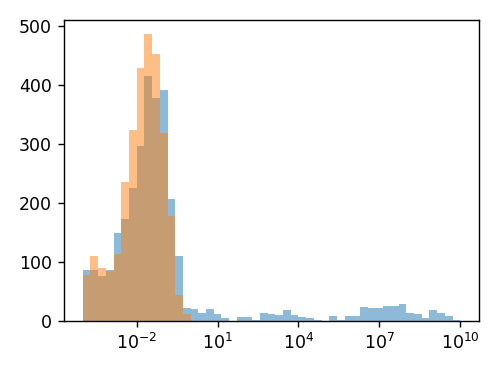:
:
array([-11.20416,  -0.94871,  -0.54481,  -0.54481,  -0.54481,   0.25607,   0.32516,   0.32516,   0.32516,   0.7408 ,   0.7408 ,   0.7408 ,   1.23234,
1.23234,   1.23234,   1.25106,   1.32904])

6.7.2. 任务 (2)¶

\begin{split}\begin{align} \frac{\partial F_{\mu \nu}}{\partial A_t} &= 2 \left( (\mu \nu | \kappa \lambda) - \frac{1}{2} (\mu \kappa | \nu \lambda) \right) \big( C_{\kappa m} C_{\lambda i} + C_{\kappa i} C_{\lambda m} \big) U_{mi}^{A_t} \\ &= \big( 4 (\mu \nu | \kappa \lambda) - (\mu \kappa | \nu \lambda) - (\mu \lambda | \kappa \nu) \big) C_{\kappa m} C_{\lambda i} U_{mi}^{A_t} \end{align}\end{split}

$\sum_{\kappa \lambda m i} \big( 2 (\mu \nu | \kappa \lambda) - (\mu \kappa | \nu \lambda) \big) C_{\kappa m} C_{\lambda i} U_{mi}^{A_t} + \sum_{\kappa \lambda m i} \big( 2 (\mu \nu | \kappa \lambda) - (\mu \kappa | \nu \lambda) \big) C_{\kappa i} C_{\lambda m} U_{mi}^{A_t}$

$\sum_{\lambda \kappa m i} \big( 2 (\mu \nu | \lambda \kappa) - (\mu \lambda | \nu \kappa) \big) C_{\lambda i} C_{\kappa m} U_{mi}^{A_t}$

$\sum_{\kappa \lambda m i} \big( 2 (\mu \nu | \kappa \lambda) + 2 (\mu \nu | \lambda \kappa) - (\mu \kappa | \nu \lambda) - (\mu \lambda | \nu \kappa) \big) C_{\kappa m} C_{\lambda i} U_{mi}^{A_t}$

$\sum_{\kappa \lambda m i} \big( 4 (\mu \nu | \kappa \lambda) - (\mu \kappa | \nu \lambda) - (\mu \lambda | \kappa \nu) \big) C_{\kappa m} C_{\lambda i} U_{mi}^{A_t}$

6.7.3. 任务 (3)¶

$\frac{\partial F_{pq}}{\partial {A_t}} = F_{pq}^{A_t} + A_{pq, mi} U_{mi}^{A_t} + F_{pm} U_{mq}^{A_t} + F_{mq} U_{mp}^{A_t}$

$\frac{\partial F_{\mu \nu}}{\partial A_t} = F_{\mu \nu}^{A_t} + A_{\mu \nu, \kappa \lambda} C_{\kappa m} C_{\lambda i} U_{mi}^{A_t}$

$\frac{\partial F_{pq}}{\partial {A_t}} = C_{\mu p} \frac{\partial F_{\mu \nu}}{\partial A_t} C_{\nu q} + F_{pm} U_{mq}^{A_t} + F_{mq} U_{mp}^{A_t}$

\begin{split}\begin{align} C_{\mu p} \frac{\partial F_{\mu \nu}}{\partial A_t} C_{\nu q} &= C_{\mu p} F_{\mu \nu}^{A_t} C_{\nu q} + C_{\mu p} C_{\nu q} A_{\mu \nu, \kappa \lambda} C_{\kappa m} C_{\lambda i} U_{mi}^{A_t} \\ &= F_{pq}^{A_t} + A_{pq, mi} U_{mi}^{A_t} \end{align}\end{split}

6.7.4. 任务 (4)¶

$\frac{\partial F_{pq}}{\partial {A_t}} = F_{pq}^{A_t} + A_{pq, mi} U_{mi}^{A_t} + F_{pm} U_{mq}^{A_t} + F_{mq} U_{mp}^{A_t}$

:
d_F_0_mo = (
+ F_1_mo
+ np.einsum("pqmi, Atmi -> Atpq", A_0_mo[:, :, :, so], to_natm_3(gradh_nr.U_1)[:, :, :, so])
+ np.einsum("pm, Atmq -> Atpq", F_0_mo, to_natm_3(gradh_nr.U_1))
+ np.einsum("mq, Atmp -> Atpq", F_0_mo, to_natm_3(gradh_nr.U_1))
)

:

:
fig, ax = plt.subplots(figsize=(4, 3))
ax.hist(abs(d_F_0_mo.ravel() - nd_F_0_mo.ravel()), bins=np.logspace(np.log10(1e-11), np.log10(1e-1), 50), alpha=0.5)
ax.hist(abs(nd_F_0_mo.ravel()), bins=np.logspace(np.log10(1e-11), np.log10(1e-1), 50), alpha=0.5)
ax.set_xscale("log")
fig.tight_layout()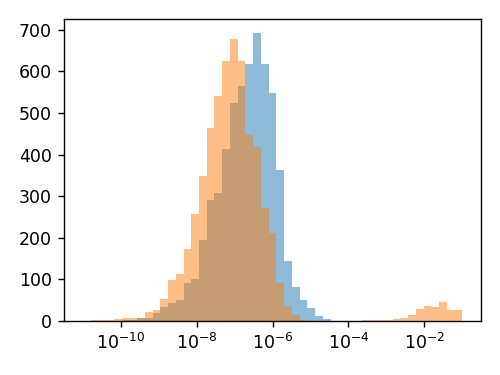:
delta_Atpq = np.eye(nmo).reshape(1, nmo, nmo).repeat(natm * 3, axis=0).reshape(natm, 3, nmo, nmo)
print("Average:", abs(d_F_0_mo * (1 - delta_Atpq)).sum() / (natm * 3 * nmo * (nmo - 1)))
print("Maximum:", np.max(d_F_0_mo * (1 - delta_Atpq)))
Average: 5.093565934419702e-07
Maximum: 2.5572817114088162e-05

:
fig, ax = plt.subplots(figsize=(4, 3))
ax.hist(abs(d_F_0_mo.diagonal(axis1=-2, axis2=-1).ravel() - nd_F_0_mo.diagonal(axis1=-2, axis2=-1).ravel()), bins=np.logspace(np.log10(1e-11), np.log10(1e-1), 50), alpha=0.5)
ax.hist(abs(nd_F_0_mo.diagonal(axis1=-2, axis2=-1).ravel()), bins=np.logspace(np.log10(1e-11), np.log10(1e-1), 50), alpha=0.5)
ax.set_xscale("log")
fig.tight_layout()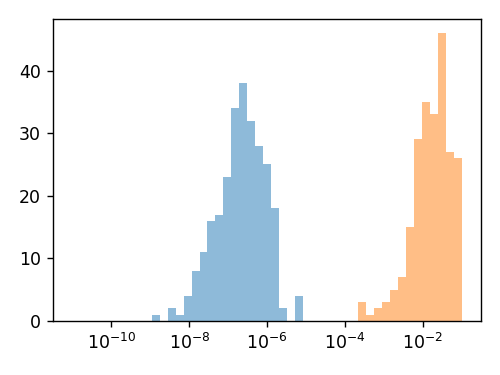6.7.5. 任务 (5)¶

$\mathtt{resp}_{\mu \nu} [X_{\kappa \lambda}^\mathbb{A}] = \big( (\mu \nu | \kappa \lambda) - \frac{1}{2} (\mu \kappa| \nu \lambda) \big) X_{\kappa \lambda}^\mathbb{A}$
:

$A_{\mu \nu, \kappa \lambda} = 4 (\mu \nu | \kappa \lambda) - (\mu \kappa | \nu \lambda) - (\mu \lambda | \kappa \nu)$

\begin{split}\begin{align} \mathtt{resp}_{\mu \nu} [X_{\kappa \lambda}^\mathbb{A} + X_{\lambda \kappa}^\mathbb{A}] &= \big( (\mu \nu | \kappa \lambda) - \frac{1}{2} (\mu \kappa| \nu \lambda) \big) X_{\kappa \lambda}^\mathbb{A} + \big( (\mu \nu | \kappa \lambda) - \frac{1}{2} (\mu \kappa| \nu \lambda) \big) X_{\lambda \kappa}^\mathbb{A} \\ &= \big( (\mu \nu | \kappa \lambda) - \frac{1}{2} (\mu \kappa| \nu \lambda) \big) X_{\kappa \lambda}^\mathbb{A} + \big( (\mu \nu | \lambda \kappa) - \frac{1}{2} (\mu \lambda| \nu \kappa) \big) X_{\kappa \lambda}^\mathbb{A} \\ &= \big( 2 (\mu \nu | \kappa \lambda) - \frac{1}{2} (\mu \kappa| \nu \lambda) - \frac{1}{2} (\mu \lambda| \nu \kappa) \big) X_{\kappa \lambda}^\mathbb{A} \\ &= \frac{1}{2} A_{\mu \nu, \kappa \lambda} X_{\kappa \lambda}^\mathbb{A} \end{align}\end{split}

\begin{split}\begin{align} A_{pq, mi} U_{mi}^\mathbb{A} &= C_{\mu p} C_{\nu q} A_{\mu \nu, \kappa \lambda} C_{\kappa m} C_{\lambda i} U_{mi}^\mathbb{A} \\ &= 2 C_{\mu p} C_{\nu q} \mathtt{resp}_{\mu \nu} [C_{\kappa m} C_{\lambda i} U_{mi}^\mathbb{A} + C_{\lambda m} C_{\kappa i} U_{mi}^\mathbb{A}] \end{align}\end{split}

$U_{\kappa \lambda}^{A_t} = C_{\kappa m} U_{mi}^{A_t} C_{\lambda i} + \mathrm{swap} (\kappa, \lambda)$
:
dmU = np.einsum("km, Atmi, li -> Atkl", C, to_natm_3(gradh_nr.U_1[:, :, so]), Co)
dmU += dmU.swapaxes(-1, -2)

:
AU_nr_resp = 2 * np.einsum("up, vq, Atuv -> Atpq", C, C, to_natm_3(resp(dmU.reshape(natm * 3, nao, nao))))

:
np.allclose(AU_nr_resp, np.einsum("pqmi, Atmi -> Atpq", A_0_mo[:, :, :, so], to_natm_3(gradh_nr.U_1[:, :, so])))
:
True

6.7.6. 任务 (6)¶

$\frac{\partial F_{pq}}{\partial \mathbb{A}} = F_{pq}^\mathbb{A} - S_{pq}^\mathbb{A} \varepsilon_q - \frac{1}{2} A_{pq, kl} S_{kl}^\mathbb{A} + A_{pq, bj} U_{bj}^\mathbb{A} + (\varepsilon_p - \varepsilon_q) U_{pq}^\mathbb{A}$

$\frac{\partial F_{pq}}{\partial \mathbb{A}} = F_{pq}^\mathbb{A} + A_{pq, mi} U_{mi}^\mathbb{A} + F_{pm} U_{mq}^\mathbb{A} + F_{mq} U_{mp}^\mathbb{A}$

$\frac{\partial F_{pq}}{\partial \mathbb{A}} \leftarrow F_{pm} U_{mq}^\mathbb{A} + F_{mq} U_{mp}^\mathbb{A} = \varepsilon_p U_{pq}^\mathbb{A} + \varepsilon_q U_{qp}^\mathbb{A}$

$\frac{\partial F_{pq}}{\partial \mathbb{A}} \leftarrow (\varepsilon_p - \varepsilon_q) U_{pq}^\mathbb{A} - S_{pq}^\mathbb{A} \varepsilon_q$

\begin{split}\begin{align} \frac{\partial F_{pq}}{\partial \mathbb{A}} \leftarrow A_{pq, mi} U_{mi}^\mathbb{A} &= A_{pq, kl} U_{kl}^\mathbb{A} + A_{pq, bj} U_{bj}^\mathbb{A} \\ &= \frac{1}{2} (A_{pq, kl} U_{kl}^\mathbb{A} + A_{pq, lk} U_{lk}^\mathbb{A}) + A_{pq, bj} U_{bj}^\mathbb{A} \\ &= \frac{1}{2} A_{pq, kl} (U_{kl}^\mathbb{A} + U_{lk}^\mathbb{A}) + A_{pq, bj} U_{bj}^\mathbb{A} \\ &= - \frac{1}{2} A_{pq, kl} S_{kl}^\mathbb{A} + A_{pq, bj} U_{bj}^\mathbb{A} \end{align}\end{split}

$\frac{\partial F_{pq}}{\partial A_t} = F_{pq}^\mathbb{A} - S_{pq}^{A_t} \varepsilon_q - \frac{1}{2} A_{pq, kl} S_{kl}^{A_t} + A_{pq, bj} U_{bj}^{A_t} + (\varepsilon_p - \varepsilon_q) U_{pq}^{A_t}$
:
d_F_0_mo_simp = (
+ F_1_mo
- S_1_mo * e
- 0.5 * Ax0_Core(sa, sa, so, so)(S_1_mo[:, :, so, so])
+ (e[:, None] - e[None, :]) * (to_natm_3(gradh_nr.U_1))
)

:
np.allclose(d_F_0_mo_simp, d_F_0_mo, atol=1e-5)
:
True

6.7.7. 任务 (7)¶

$\begin{split}\begin{equation} \left\{ \begin{matrix} U_{ab}^\mathbb{A} &= - \frac{B_{ab}^\mathbb{A} + A_{ab, ck} U_{ck}^\mathbb{A}}{\varepsilon_a - \varepsilon_b} &\quad (a \neq b) \\ U_{aa}^\mathbb{A} &= - \frac{1}{2} S_{aa}^\mathbb{A} \end{matrix} \right. \end{equation}\end{split}$
:
U_1_vv = - (B_1[:, :, sv, sv] + Ax0_Core(sv, sv, sv, so)(U_1_ai)) / (ev[:, None] - ev[None, :])
for p in range(nocc, nmo):
U_1_vv[:, :, p - nocc, p - nocc] = - S_1_mo[:, :, p, p] / 2
U_1_vv.shape
:
(4, 3, 13, 13)

: